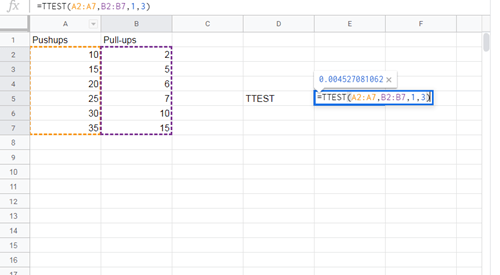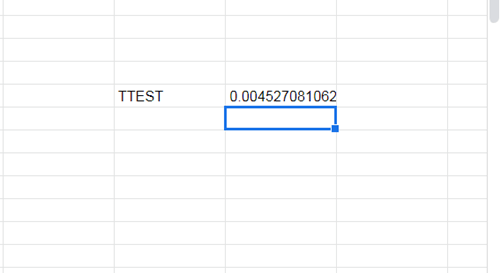# How To Calculate p-Value in Google Sheets

The p-value is one of the most important concepts in statistics. When working on research projects, this is the output data scientists most often rely on.This article will show you everything you need to know about the topic. By the end of the article, you will be able to easily calculate the p-value and check your results.

## What Is the p-Value?

The p-value is used to determine whether certain hypotheses are correct or not. Basically, scientists will choose a value, or range of values, which express the normal, expected result when data isn’t correlated. After calculating the p-value of their data sets, they will know just how close they are to these results.

The constant that represents the expected results is called the significance level. Although you can choose this number based on previous research, it is usually set to 0.05.

If the calculated p-value is way below the significance level, then the expected result did not come to pass. The lower the p-value, the more likely it is that your data expresses some kind of correlation.

## How Do You Calculate the p-Value Manually?

These are the steps for calculating the p-value on paper:

1. Determine the expected results for your experiment.
2. Calculate and determine the observed results for your experiment.
3. Determine the degree of freedom – how much deviation from the respected results counts as significant?
4. Compare the first, expected results to the observer results with a chi-square.
5. Choose the significance level.
6. Approximate your p-value by using the chi-square distribution table.
7. Reject or keep your starting null hypothesis.

As you can see, there is quite a lot to calculate and take into consideration when doing this with pen and paper. You would need to check if you have followed the correct formulas for all the steps, as well as double-check whether you have the correct values.

To avoid the risk of ending up with false results due to bad calculations, it is best to use tools like Google Sheets. Since the p-value is so significant, the developers have included a function that will calculate it directly. The following section will show you how to do it.

## Calculating the p-Value in Google Sheets

The best way to explain this would be through an example that you can follow. If you already have an existing table, simply apply what you learn from the following tutorial.

We will start by making two sets of data. After that, we will compare the created data sets to see if there’s a statistical significance between them.

Let’s say that we need to examine data for a personal trainer. The personal trainer provided us with their client’s numbers regarding their pushup and pull-up progression, and we have entered them into Google Spreadsheet.The table is very basic but it will serve for the purposes of this article.

In order to compare these two different sets of data, we will need to use Google Spreadsheet’s T-TEST function.

This function’s syntax looks like this: TTEST(array1,array2,tails,type) but you can also use the syntax T.TEST(array1,array2,tails,type) – both refer to the same function.

Array1 is the first data set. In our case, that would be the entire Pushups column (except for the column name, of course).

Array2 is the second data set, which is everything under the Pull-ups column.

Tails represent the number of tails that are used for the distribution. You only have two options here:

1 – one-tailed distribution

2 – two-tailed distribution

Type represents an integer value which can be 1 (paired T-TEST), 2 (two-sampled equal variance T-Test), or 3 (two-sample unequal variance T-Test).

We will name a column of our choosing TTEST and display this function’s results in the column next to it.

To use this function, simply click on the empty column where you want the p-values to be displayed, and enter the formula that you need. For our example, we will enter the following formula: =TTEST(A2:A7,B2:B7,1,3). As you can see, A2:A7 signifies the starting and ending point of our first column. You can simply hold your cursor at the first position (A2) and drag it to the bottom of your column and Google Spreadsheets will automatically update your formula. Add a comma to your formula and do the same thing for the second column as well.

Then just fill in the tails and type arguments (separated by commas) and hit Enter.

Your result should appear in the column where you have typed the formula.## Common Error Messages

If you have made a mistake typing your TTEST formula, you have probably seen one of these error messages:

1. #N/A – displayed if your two data sets have different lengths.
2. #NUM – displayed if the entered tails argument isn’t equal to 1 or 2. It can also be displayed if the type argument isn’t equal to 1, 2, or 3.
3. #VALUE! – displayed if you have entered non-numeric values for tails or type arguments.